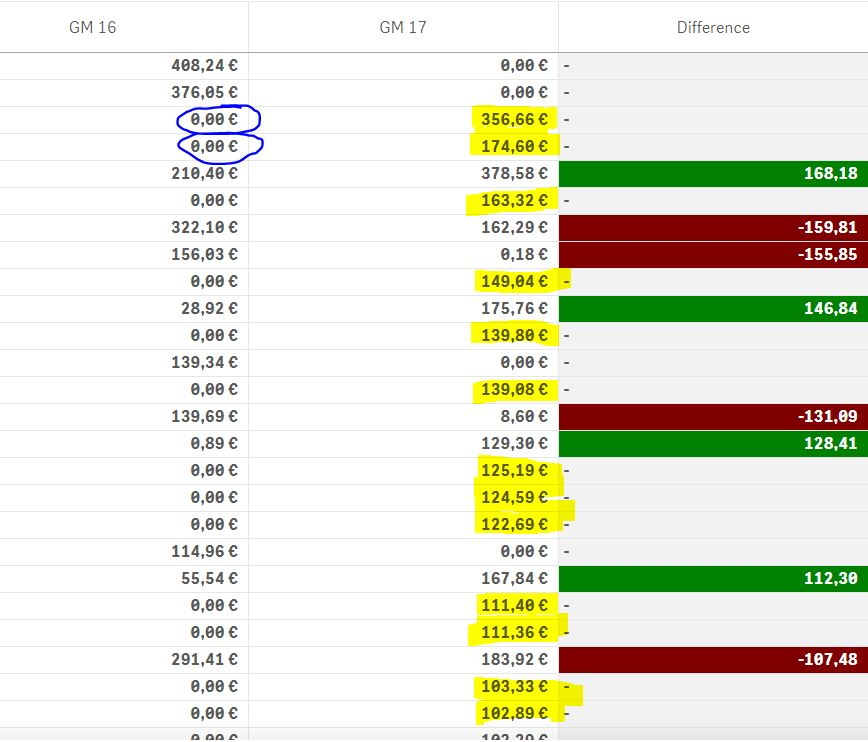# Connectivity & Data Prep

Announcements
Our next Qlik Insider session will cover new key capabilities. Join us August 11th REGISTER TODAY
cancel
Showing results for
Did you mean:Contributor III

## Difference & % difference

Hello,

Can you please help me in getting the correct difference & the % difference of two numbers (column GM 2016; column GM 2017),

I want to obtain the difference only to non 0 number that are present in both columns.

I used "fabs(Sum({\$<[Year]={"2016"}>}[Gross Margin Actual Year])) - (Sum({\$<[Year]={"2017"}>}[Gross Margin Actual Year]))"

to get the difference as the data in excel is in one column.

Sachin

10 RepliesMVP

May be this

If(fabs(Sum({\$<[Year]={"2016"}>}[Gross Margin Actual Year])) <> 0 and fabs(Sum({\$<[Year]={"2017"}>}[Gross Margin Actual Year])) <> 0, fabs(Sum({\$<[Year]={"2016"}>}[Gross Margin Actual Year])) - (Sum({\$<[Year]={"2017"}>}[Gross Margin Actual Year])))

Basically check if part 1 of the expression is not 0 and part 2 is not zero, then perform the calculationContributor III
Author

Hello Sunny,

Thank you for your response, it worked well.

I have one more question.

I want to create a bar chart and show only the numbers that are zero in GM 2016. This means the numbers are only in GM 2017 ( not the numbers that are present in both). As shown in figure below.I tried to use this formula but i failed.

If(fabs(Sum({\$<[Year]={"2016"}>}[Gross Margin Actual Year])) <> 0 , Sum({\$<[Year]={"2017"}>}[Gross Margin Actual Year]) ,0)

Thank you

SachinMVP

May be this

If(fabs(Sum(TOTAL {\$<[Year]={"2016"}>}[Gross Margin Actual Year])) = 0 , Sum({\$<[Year]={"2017"}>}[Gross Margin Actual Year]), 0)Contributor III
Author

This doesnot work,

It gives all rows that are 0MVP

Then this?

If(fabs(Sum(TOTAL {\$<[Year]={"2016"}>}[Gross Margin Actual Year])) > 0 , Sum({\$<[Year]={"2017"}>}[Gross Margin Actual Year]), 0)Contributor III
Author

No, i have tried this and this gives the results of both 2016 & 2017 non zero numbers.Contributor III
Author

also tried this

If(fabs(Sum(TOTAL [Gross Margin Actual Year])) <> 0 , Sum({\$<[Year]={"2017"}>}[Gross Margin Actual Year]), 0)

and this gives all the values of 2017 (which are in 2016 & 2017, but the numbers of 2017). But what i need is the values of only 2017 that means it may be new row or 2016 = 0MVP

Can you, once again, put it in words as to what you are looking to get?Contributor III
Author

the column GM 16 & GM 17 are in one column in excel. so I want to a bar graph that shows only the numbers of GM 17 which are highlighted in yellow. The criteria here is that these numbers are zero in 2016.  So I want thee numbers in graph not the ones that are in both the columns.Community Browser